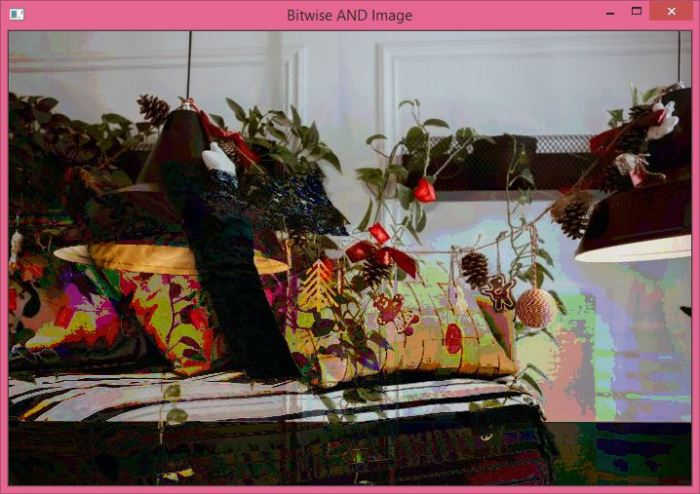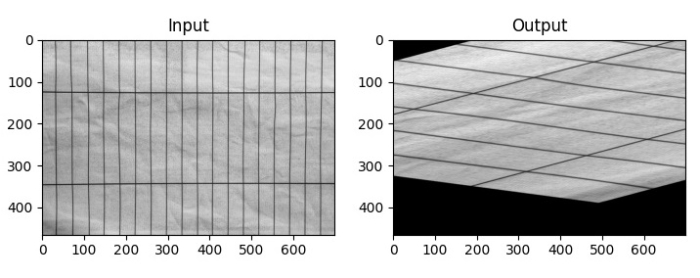# How to perform bitwise AND operation on two images in OpenCV Python?

A very important application of bitwise AND operation in computer vision or image processing is for creating masks of the image. We can also use the operator to add watermarks to an image.

The pixel values of an image are represented as a numpy ndarray. The pixel values are stored using 8-bit unsigned integers (uint8) in a range from 0 to 255. The bitwise AND operation between two images is performed on the binary representation of these pixel values of corresponding images.

Given below is the syntax to perform bitwise AND operation on two images −

cv2.bitwise_and(img1, img2, mask=None)


img1 and img2 are the two input images and mask is a mask operation.

## Steps

To compute bitwise AND between two images, you can follow the steps given below −

Import the required library OpenCV. Make sure you have already installed it.

import cv2


Read the images using cv2.imread() method. The width and height of images must be the same.

img1 = cv2.imread('lamp.jpg')


Compute the bitwise AND on the images using cv2.biwise_and(img1, img2).

and_img = cv2.bitwise_and(img1,img2)


Display the bitwise AND image.

cv2.imshow('Bitwise AND Image', and_img)
cv2.waitKey(0)
cv2.destroyAllWindows()


## Input Image

We will use the following images as the input files in the examples below.## Example 1

In the following Python program, we compute the bitwise AND on the two color images −

# import required libraries
import cv2

# The size of both images must be the same.

# compute bitwise AND on both images
and_img = cv2.bitwise_and(img1,img2)

# display the computed bitwise AND image
cv2.imshow('Bitwise AND Image', and_img)
cv2.waitKey(0)
cv2.destroyAllWindows()


## Output

When you run this Python program, it will produce the following output −## Example 2

The python program below shows the application of bitwise AND operation on images. We created a mask and then used to perform the bitwise_and operation.

# import required libraries
import cv2
import matplotlib.pyplot as plt
import numpy as np

# Read an input image as a gray image

# compute the bitwise AND using the mask

# display the input image, mask, and the output image
plt.subplot(221), plt.imshow(img, 'gray'), plt.title("Original Image")